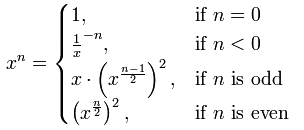Category
Categories
Sort By
• Latest
• Year

# Java Bitwise Operator using efficiently to improve Performance

26 / May / 2016 by Sandeep Gupta 0 comments

Many of us know the various operators available in Java. But do we really use them all efficiently. Here I am illustrating one simple example which can show the high performance gain with bitwise operatator than the standard solution.

Lets calculate the mathematical expression xi.e. x’s power n.

To solve this problem anyone can easily say its just one loop with n times and adding a in that loop. Thats very simple and fast. Really? Lets analyze it, we have just used O(n) complexity to find the result which means 820 will take 20 loop iterations to calculate it.

```xn = x * x ...... * x (n times)
```

Do we really need so much iterations to calculate it?

Following mathematical formula can help us to reduce the O(n) complexity to O(log n) complexityBitwise operator can be used to achieve this by shifting the bits of power. Following sample code is self explainatory.

[java]
public class PowerAofB {
public static void main(String[] args) {
// Basic way with O(n)
int a = 9;
int b = 12;
long result = 1;
for (int i = 1; i <= b; i++) {
result *= a;
}
System.out.println(result);

// Efficient way with O(log n)
System.out.println(ipow(a,b));
}

private static long ipow(int base, int exp)
{
long result = 1;

while (exp != 0)
{
if ((exp & 1) == 1){
result *= base;
}
//right shifting bytes with sign 1 position
//equivalent of division of 2
exp >>= 1;
base *= base;
}

return result;
}
}
[/java]

Comparison between basic approach and new approach

 Approach Example iteration count Execution time (nano seconds) Basic Way 1215 15 1140 Using Bitwise Operator 1215 5 570 Basic Way 4122 122 4561 Using Bitwise Operator 4122 8 1710

Image Reference: http://www.programminglogic.com/fast-exponentiation-algorithms/

FOUND THIS USEFUL? SHARE IT

### Tag -

Complexity Java Operators performanceServices